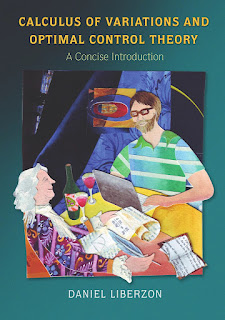CALCULUS MATHEMATICS

# Calculus of Variations and Optimal Control Theory A Concise Introduction Free DownloadThis textbook offers a concise yet rigorous introduction to calculus of variations and optimal control theory, and is a self-contained resource for graduate students in engineering, applied mathematics, and related subjects. Designed specifically for a one-semester course, the book begins with calculus of variations, preparing the ground for optimal control. It then gives a complete proof of the maximum principle and covers key topics such as the Hamilton-Jacobi-Bellman theory of dynamic programming and linear-quadratic optimal control. Calculus of Variations and Optimal Control Theory also traces the historical development of the subject and features numerous exercises, notes and references at the end of each chapter, and suggestions for further study.